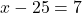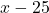## Write as a mathematical equation. If 25 is subtracted from a number, the result is 7.

Question

Write as a mathematical equation.
If 25 is subtracted from a number, the result is 7.

in progress 0
4 months 2021-09-05T17:02:27+00:00 2 Answers 90 views 0

1. x-25=7
x=32

Now double check:
32-25=7First, we must understand the operation occurring. The sentence clearly tells us that we are subtracting. The next important step is finding out the order. In the equation 25 is being taken away from the number; this means that the equation should be. Then, it says that the result is 7. This means the expression should equal 7.